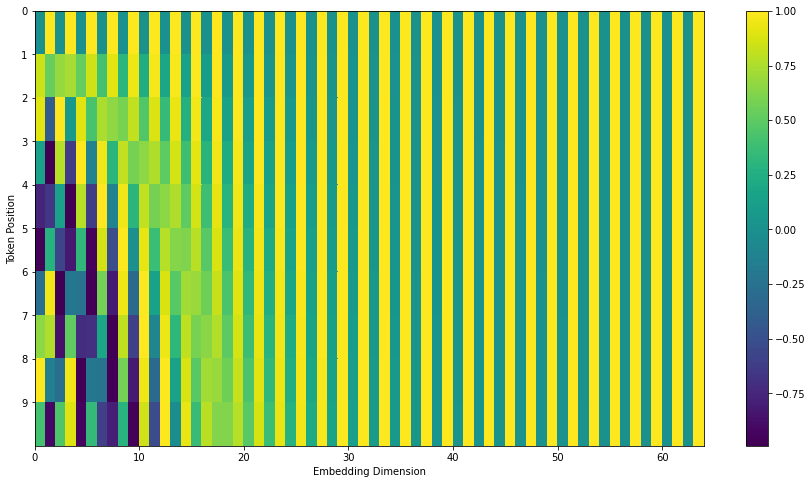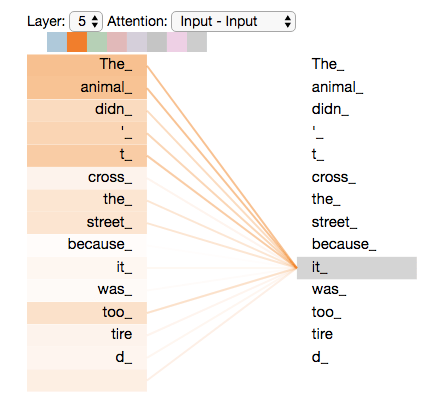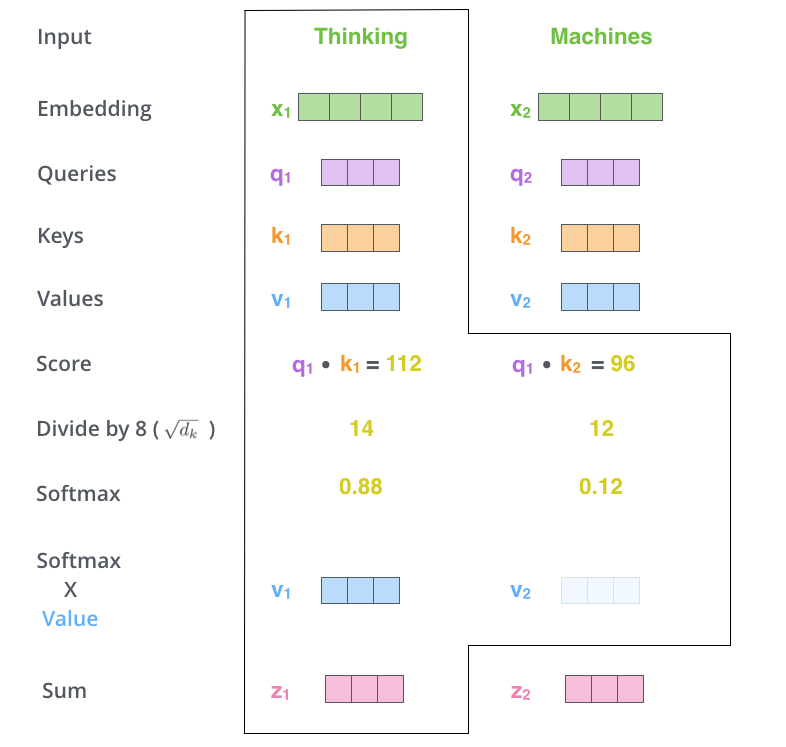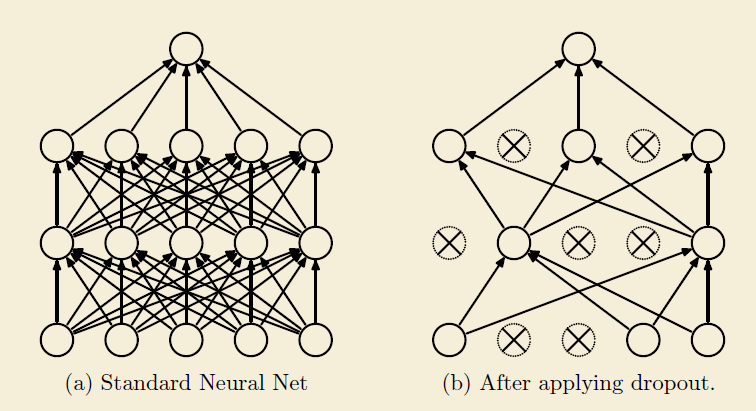# 全网最强ViT (Vision Transformer)原理及代码解析

今天我们来详细了解一下Vision Transformer。基于timm的代码。1. Patch Embedding

Transformer原本是用来做NLP的工作的，所以ViT的首要任务是将图转换成词的结构，这里采取的方法是如上图左下角所示，将图片分割成小块，每个小块就相当于句子里的一个词。这里把每个小块称作Patch，而Patch Embedding就是把每个Patch再经过一个全连接网络压缩成一定维度的向量。

``````# 默认img_size=224, patch_size=16，in_chans=3，embed_dim=768，
self.patch_embed = embed_layer(
img_size=img_size, patch_size=patch_size,
in_chans=in_chans, embed_dim=embed_dim)``````

``````class PatchEmbed(nn.Module):
""" 2D Image to Patch Embedding
"""
def __init__(self, img_size=224, patch_size=16, in_chans=3, embed_dim=768, norm_layer=None, flatten=True):
super().__init__()
# img_size = (img_size, img_size)
img_size = to_2tuple(img_size)
patch_size = to_2tuple(patch_size)
self.img_size = img_size
self.patch_size = patch_size
self.grid_size = (img_size // patch_size, img_size // patch_size)
self.num_patches = self.grid_size * self.grid_size
self.flatten = flatten
# 输入通道，输出通道，卷积核大小，步长
# C*H*W->embed_dim*grid_size*grid_size
self.proj = nn.Conv2d(in_chans, embed_dim, kernel_size=patch_size, stride=patch_size)
self.norm = norm_layer(embed_dim) if norm_layer else nn.Identity()

def forward(self, x):
B, C, H, W = x.shape
assert H == self.img_size and W == self.img_size, \
f"Input image size ({H}*{W}) doesn't match model ({self.img_size}*{self.img_size})."
x = self.proj(x)
if self.flatten:
x = x.flatten(2).transpose(1, 2)  # BCHW -> BNC
x = self.norm(x)
return x``````

proj虽然用的是卷积的写法，但其实是将每个patch接入了同样的全连接网络，将每个patch转换成了一个向量。x的维度是（B，C，H，W）其中B是batch size，C通常是三通道，H和W分别是图片的高和宽，而输出则是（B，N，E），B依然是batch size，N则是每张图被切割成了patch之后，patch的数量，E是embed_size，每个patch会通过一个全连接网络转换成一个向量，E是这个向量的长度，根据卷积的原理，也可以理解为每个patch的特征数量。

2. Positional Encoding

把图片分割成了patch，然后把每个patch转换成了embedding，接下来就是在embedding中加入位置信息。产生位置信息的方式主要分两大类，一类是直接通过固定算法产生，一种是训练获得。但加位置信息的方式还是比较统一且粗暴的。pos是单词在句子中的位置，或者patch在图中的位置，而i对应的是embedding的位置，dmodel对应的是patch embedding的长度，。这里说一下为什么要加这个位置编码，以及加上以后会有什么效果，我们观察上两幅图，可以发现，位置编码是随位置而改变的，位置差别越大的，那么向量差别也越大。在NLP课程里说过，把一个词转换成向量，就好像把一个词映射到了一个高维空间的位置，意思相近的词会在高维空间内比较靠近，而加上位置向量，会让位置相近的词更靠近，位置远的词离得更远。再来，为什么用cos，sin这种方式，作者的解释是，使用sin和cos编码可以得到词语之间的相对位置。3. Self-Attention

接下来看ViT中的Attention，这和Transformer中的self-attention应该是一致的，我们先看看参考是如何介绍self-attention的。参考举了一个语义处理的例子，有一句话是这样的

“The animal didn't cross the street because it was too tired.”Self-attention就是在这种需求下产生的，如上图所示，我们应当有一个结构能够表达每个单词和其他每个单词的关系。那这里我们处理的是图像问题，Self-attention的存在就可以理解成，我们应当有一个结构能够表达每个patch和其他patch的关系。之前说过，图像中的patch和语义处理中的词可以同等来看。

我们再来看具体怎么实现的：

1. 基于输入向量创建三个向量：query向量，key向量和value向量。2. 由query向量和key向量产生自注意力。Thinking和Machine可以理解为图片被切分的两个patch，现在计算Thinking的自注意力，通过q乘k，除以一定系数（scaled dot-product attention，点积得到的结果值通常很大，使得softmax结果不能很好地表达attention值。这时候除以一个缩放因子，可以一定程度上减缓这种情况。），通过softmax之后会得到一个关于Thinking的注意力向量，比如这个例子是[0.88, 0.12]，这个向量的意思是，要解释Thinking这个词在这个句子中的意思，应当取0.88份Thinking原本的意思，再取0.12份Machine原本的意思，就是Thinking在这个句子中的意思。最后图中Sum之后的结果所表达的就是每个单词在这个句子当中的意思。整个过程可以用下面这张图表达：``````class Attention(nn.Module):
def __init__(self, dim, num_heads=8, qkv_bias=False, attn_drop=0., proj_drop=0.):
super().__init__()
# q,k,v向量长度
self.qkv = nn.Linear(dim, dim * 3, bias=qkv_bias)
self.attn_drop = nn.Dropout(attn_drop)
self.proj = nn.Linear(dim, dim)
self.proj_drop = nn.Dropout(proj_drop)

def forward(self, x):
# 这里C对应上面的E，向量的长度
B, N, C = x.shape
qkv = self.qkv(x).reshape(B, N, 3, self.num_heads, C // self.num_heads).permute(2, 0, 3, 1, 4)
# 这里的效果是从每个向量产生三个向量，分别是query，key和value
q, k, v = qkv.unbind(0)   # make torchscript happy (cannot use tensor as tuple)
attn = (q @ k.transpose(-2, -1)) * self.scale
attn = attn.softmax(dim=-1)
attn = self.attn_drop(attn)
x = (attn @ v).transpose(1, 2).reshape(B, N, C)
# (B, N, C) -> (B, N, C)
x = self.proj(x)
x = self.proj_drop(x)
return x``````5. Layer Normalization

Layer normalization对应的一个概念是我们熟悉的Batch Normalization，这两个根本的不同在于，Layer normalization是对每个样本的所有特征进行归一化，而Batch Normalization是对每个通道的所有样本进行归一化。

为了便于理解，这里贴一下官网给LN的示例代码：

``````# NLP Example
batch, sentence_length, embedding_dim = 20, 5, 10
embedding = torch.randn(batch, sentence_length, embedding_dim)
# 指定归一化的维度
layer_norm = nn.LayerNorm(embedding_dim)
# 进行归一化
layer_norm(embedding)

# Image Example
N, C, H, W = 20, 5, 10, 10
input = torch.randn(N, C, H, W)
# Normalize over the last three dimensions (i.e. the channel and spatial dimensions)
# as shown in the image below
layer_norm = nn.LayerNorm([C, H, W])
output = layer_norm(input)``````6. Drop Path

Dropout是最早用于解决网络过拟合的方法，是所有drop类方法的始祖。方法示意图如下：接下来以dropout的思路来理解drop path，drop path没找到示意图，那直接看timm上的代码：

``````def drop_path(x, drop_prob: float = 0., training: bool = False):
if drop_prob == 0. or not training:
return x
# drop_prob是进行droppath的概率
keep_prob = 1 - drop_prob
# work with diff dim tensors, not just 2D ConvNets
# 在ViT中，shape是(B,1,1),B是batch size
shape = (x.shape,) + (1,) * (x.ndim - 1)
# 按shape,产生0-1之间的随机向量,并加上keep_prob
random_tensor = keep_prob + torch.rand(shape, dtype=x.dtype, device=x.device)
# 向下取整，二值化，这样random_tensor里1出现的概率的期望就是keep_prob
random_tensor.floor_()  # binarize
# 将一定图层变为0
output = x.div(keep_prob) * random_tensor
return output``````

7. Encoder

Transformer的架构图：Transformer是由一堆encoder和decoder形成的，那encoder一般的架构图如下：Encoder在ViT中的实现细节如下面代码所示（layer normalization -> multi-head attention -> drop path -> layer normalization -> mlp -> drop path），换了个名字，叫block了：

``````class Block(nn.Module):

def __init__(self, dim, num_heads, mlp_ratio=4., qkv_bias=False, drop=0., attn_drop=0.,
drop_path=0., act_layer=nn.GELU, norm_layer=nn.LayerNorm):
super().__init__()
# 将每个样本的每个通道的特征向量做归一化
# 也就是说每个特征向量是独立做归一化的
# 我们这里虽然是图片数据，但图片被切割成了patch，用的是语义的逻辑
self.norm1 = norm_layer(dim)
# NOTE: drop path for stochastic depth, we shall see if this is better than dropout here
self.drop_path = DropPath(drop_path) if drop_path > 0. else nn.Identity()
self.norm2 = norm_layer(dim)
mlp_hidden_dim = int(dim * mlp_ratio)
# 全连接，激励，drop，全连接，drop,若out_features没填，那么输出维度不变。
self.mlp = Mlp(in_features=dim, hidden_features=mlp_hidden_dim, act_layer=act_layer, drop=drop)

def forward(self, x):
# (B, N, C) -> (B, N, C)
x = x + self.drop_path(self.attn(self.norm1(x)))
# (B, N, C) -> (B, N, C)
x = x + self.drop_path(self.mlp(self.norm2(x)))
return x``````

``````# stochastic depth decay rule
dpr = [x.item() for x in torch.linspace(0, drop_path_rate, depth)]
self.blocks = nn.Sequential(*[
Block(
attn_drop=attn_drop_rate, drop_path=dpr[i], norm_layer=norm_layer, act_layer=act_layer)
for i in range(depth)])``````

8. Forward Features

Patch embedding -> 加cls -> 加pos embedding -> 用blocks进行encoding -> layer normalization -> 输出图的embedding

``````def forward_features(self, x):
# x由（B，C，H，W）->（B，N，E）
x = self.patch_embed(x)
# stole cls_tokens impl from Phil Wang, thanks
# cls_token由(1, 1, 768)->(B, 1, 768), B是batch_size
cls_token = self.cls_token.expand(x.shape, -1, -1)
# dist_token是None,DeiT models才会用到dist_token。
if self.dist_token is None:
# x由(B, N, E)->(B, 1+N, E)
x = torch.cat((cls_token, x), dim=1)
else:
# x由(B, N, E)->(B, 2+N, E)
x = torch.cat((cls_token, self.dist_token.expand(x.shape, -1, -1), x), dim=1)
# +pos_embed:(1, 1+N, E)，再加一个dropout层
x = self.pos_drop(x + self.pos_embed)
x = self.blocks(x)
# nn.LayerNorm
x = self.norm(x)
if self.dist_token is None:
# 不是DeiT，输出就是x[:,0]，(B, 1, 768)，即cls_token
return self.pre_logits(x[:, 0])
else:
# 是DeiT，输出就是cls_token和dist_token
return x[:, 0], x[:, 1]``````

9. Forward

``````    def forward(self, x):
#（B，C，H，W）-> (B, 1, 768)
# (B,C,H,W) -> (B, 1, 768), (B, 1, 768)
x = self.forward_features(x)

# 如果num_classes>0, (B, 1, 768)->(B, 1, num_classes)
# 否则不变
if self.training and not torch.jit.is_scripting():
return x, x_dist
else:
# during inference,
# return the average of both classifier predictions
return (x + x_dist) / 2
else:
# 如果num_classes>0, (B, 1, 768)->(B, 1, num_classes)
# 否则不变
return x``````

 Jay Alammar, The Illustrated Transformer, jalammar.github.io, 2018

 简枫，聊聊 Transformer，知乎，2019

 大师兄，模型优化之Layer Normalization，知乎，2020

 TensorFlow Core，理解语言的 Transformer 模型，TensorFlow，https://www.tensorflow.org/tutorials/text/transformer觉得有用，关注，在看，点赞，转发分享来一波哦！